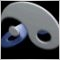# IF Statement Problem428

Hi,

Can anybody help me understand that the following if statment is not working???

double Minimum = lowest of last N candles;int ShortRSI=X;

if(iRSI(NULL,0,14,MODE_CLOSE,1)<=ShortRSI && Bid<Minimum )
{
ticket=OrderSend(Symbol(),OP_SELL,.................

If I remove && part of the IF statment, then it works okay.

Any clues

ckmoied2646

Maybe you are including the current Bid in the calculation of Minimum, so Bid cannot be < Minimum.428

ok so here is the problem

if(iRSI(NULL,0,14,MODE_CLOSE,1)>=LongRSI && Ask>Maximum) = Works Fine

if(iRSI(NULL,0,14,MODE_CLOSE,1)<=ShortRSI && Bid<Minimum) = Doesn't work

if(iRSI(NULL,0,14,MODE_CLOSE,1)>=LongRSI && Close>Maximum) = Doesn't work

if(iRSI(NULL,0,14,MODE_CLOSE,1)<=ShortRSI && Close<Minimum) = Doesn't work

if(iRSI(NULL,0,14,MODE_CLOSE,1)>=LongRSI && iClose(Symbol(),PERIOD_H1,1)>Maximum) = Doesn't work

if(iRSI(NULL,0,14,MODE_CLOSE,1)<=ShortRSI && iClose(Symbol(),PERIOD_H1,1)<Minimum) = Doesn't work

where as LongRSI=60, ShortRSI=40, Maximum/Minimum are the highest and lowest values of hte last N bars.

Its causing me a big headache.

Any support would be much appreciated.2646

" Its causing me a big headache"

Debug the code then. Print out the values that you are comparing and figure out what is wrong.

Go step by step until you find what is not coded according to your plan.

Your statement "Doesn't work" actually means "This doesn't give the result I expected with the values I have generated, so either the logic of this operation or the values it is operating upon may be erroneous"